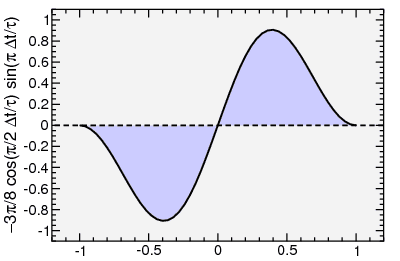## Thursday, January 7, 2010

### vibrational modes and filtering

Last post, I discussed how road vibration transmits a spectrum of acceleration to the frame, which excites oscillation modes in the relatively complex bike-rider system. MIT measurements showed oscillations near 30 Hz (of lesser magnitude, less in carbon fiber than in steel and Al) and near 50 Hz (of greater magnitude, more in Al than in carbon fiber or steel).

I generated simulated noise spectra with, successively, a direct ground noise component at 0 Hz, then a mode at 30 Hz, then an additional mode at 50 Hz. The magnitudes of the latter two modes were based on the MIT data. Here's the curves. As you can see, the 30 Hz and 50 Hz modes have a large effect on the acceleration:simulated vibration data in frame, adding modes

Now suppose I was trying to extract cadence from such accelerometer data. All of this would be considered "noise": not part of a ±1 g oscillation as the gravity vector spins in a circle relative to spindle coordinates. Essentially I want the noise magnitude to be reduced to less than ±1g, with as few "wiggles" as possible.

Pedaling at 180 rpm, a very high cadence by any standard, results in the pedals rotating at 3 Hz: every 0.33 seconds. So it seems reasonable filtering these data with a filter with a time constant sufficiently smaller than 0.33 seconds should remove the unwanted vibrations without eliminating the signal associated with the rotating pedals.

Recall I spent several (probably too many) posts describing my experiments in digital filtering. In particular a cos(π/2 t/τ) sin(πt/τ) convolution function cut off low-frequency signal components (proportional to frequency) as well as high frequency signal components (proportional to the square of frequency). The shape of the filter is as follows:Convolution function for data smoothing

I'll apply this filter with τ = 0.15 seconds: more than a factor of two less than the time it takes for a pedal revolution at 180 rpm, but long enough that it should essentially eliminate the influence of these 30 Hz and 50 Hz modes. Note this is not the causal version of the filter: that's not important, the difference in the causal version is just a delay of τ.

So I did that. I plot in the following both the smoothed vibration and the smoothed net signal from the vibrations superposed on a sinusoidally varying gravity signal from a pedal rotating at 180 rpm. Note the filter reduced the ±1 g gravity signal to ±0.8 g.Application of filter to vibration data, gravity relative to rotating spindle, and the sum

Only one vibration curve is shown. This is because only the ground noise contributes. To the pixel resolution of the plot, the 30 Hz and 50 Hz components have been completely eliminated by the filter. There's some subtlety with filtering sampled data where the sampling rate is less than the so-called Nyquist rate. But I'll skip that. I assume the sampling is done correctly.

So with this, if I wanted to measure rpm's by finding zero-crossings of an acceleration component, you can see the filtered vibrations shift the locations of the zero crossings a bit (which adds noise to a cadence measurement). But the number of zero crossings (at least on this limited data sample) is not changed. In contrast, trying to count zero crossings in the presence of the unfiltered vibration signal would have been completely hopeless.

At very low cadences, for example less than 30 Hz, the ground noise may be a bigger problem. There's tricks one can perform to substantially reduce the problem. But that's too much detail. At least the internal bike modes (30 Hz, 50 Hz) clearly are not an issue once the data has been appropriately smoothed.